# Visualizing search keyword overlap

The other day someone asked me to look at the search data for Debug Pest Control, a pest management company based in Rhode Island. One of the things I wanted to visualize was how the search terms overlapped with each other.

To do this, I created a graph where the nodes are the keywords and edges join nodes that share a word. (A “keyword” is actually a set of words, whatever someone types into a search.) The result was a solid gray blob be cause the nodes are so densely connected.

I thinned the graph by first only looking at keywords that have occurred at least twice. Then I made my connection criteria a little stronger. I take the union of the individual words in two keywords and create a connection if at least 1/3 of these words appear in both keywords.(You can click on the image to see a larger version.)

The area of each red dot is proportional to the number of times someone has come to the site using that keyword. You can see that there’s long-tail behavior: while some dots are much larger than others, there are a lot of little dots.

In case you’re curious, these are the top keywords:

1. pest control
2. ri
3. pest control ri
4. pest control rhode island,
5. plants that keep bees away
6. poisonous snakes in ri
7. plants that keep bees and wasps away
8. plants to keep bees away
9. plants that keep wasps away
10. pest control companies

The keywords were filtered a little before making the graph. Some of the top keywords contained “debug.” These may have been people using the term to refer to eliminating pests from their property, but more likely they were people who knew the company name. By removing “debug” from each of the keywords, the results focus on people searching for the company’s services rather than the company itself.

# Spectral coordinates in Python

A graph doesn’t have any geometric structure unless we add it. The vertices don’t come with any position in space. The same graph can look very different when arranged different ways.

Spectral coordinates are a natural way to draw a graph because they are determined by the properties of the graph, not arbitrary aesthetic choices. Construct the Laplacian matrix and let x and y be the eigenvectors associated with the second and third eigenvalues. (The smallest eigenvalue is always zero and has an eigenvector of all 1’s. The second and third eigenvalues and eigenvectors are the first to contain information about a graph.) The spectral coordinates of the ith node are the ith components of x and y.

We illustrate this with a graph constructed from a dodecahedron, a regular solid with twenty vertices and twelve pentagonal faces. You can make a dodecahedron from a soccer ball by connecting the centers of all the white hexagons. Here’s one I made from Zometool pieces for a previous post: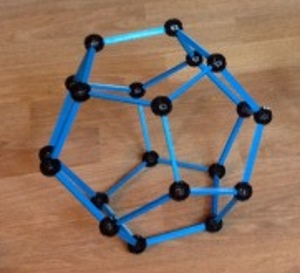Although we’re thinking of this graph as sitting in three dimensions, the nodes being the corners of pentagons etc., the graph simply says which vertices are connected to each other. But from this information, we can construct the graph Laplacian and use it to assign plane coordinates to each point. And fortunately, this produces a nice picture: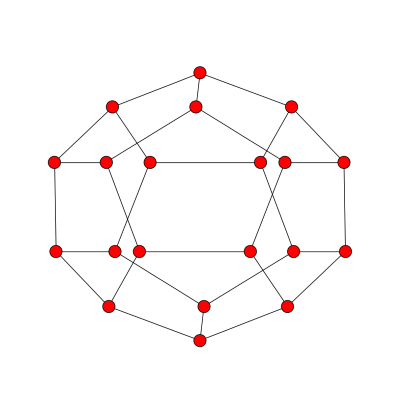Here’s how that image was created using Python’s NetworkX library.

    import networkx as nx
import matplotlib.pyplot as plt
from scipy.linalg import eigh

# Read in graph and compute the Laplacian L ...

# Laplacian matrices are real and symmetric, so we can use eigh,
# the variation on eig specialized for Hermetian matrices.
w, v = eigh(L) # w = eigenvalues, v = eigenvectors

x = v[:,1]
y = v[:,2]
spectral_coordinates = {i : (x[i], y[i]) for i in range(n)}
G = nx.Graph()

nx.draw(G, pos=spectral_coordinates)
plt.show()


Update: After posting this I discovered that NetworkX has a method draw_spectral that will compute the spectral coordinates for you.

# Spectra of complete graphs, stars, and rings

A few examples help build intuition for what the eigenvalues of the graph Laplacian tell us about a graph. The smallest eigenvalue is always zero (see explanation in footnote here).

For a complete graph on n vertices, all the eigenvalues except the first equal n. The eigenvalues of the Laplacian of a graph with n vertices are always less than or equal to n, this says the complete graph has the largest possible eigenvalue. This makes sense in light of the previous post: adding edges increases the eigenvalues. When you add as many edges as possible, you get the largest possible eigenvalues.Conversely, if all the non-zero eigenvalues of the graph Laplacian are equal, the graph is complete.

Next we look at the case of a star with n-1 vertices connected to a central vertex. The smallest eigenvalue is zero, as always, and the largest is n. The ones in the middle are all 1. In particular, the second eigenvalue, the algebraic connectivity, is 1. It’s positive because the graph is connected, but it’s not large because the graph is not well connected: if you remove the central vertex it becomes completely disconnected.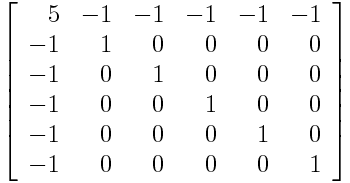Finally, we look at a cyclical graph, a ring with n vertices. Here the eigenvalues are 2 – 2 cos(2πk/n) where 0 ≤ kn/2. In particular, smallest non-zero eigenvalue is 2 – 2 cos(2π/n) and so as n increases, the algebraic connectivity approaches zero. This is curious since the topology doesn’t change at all. But from a graph theory perspective, a big ring is less connected than a small ring. In a big ring, each vertex is connected to a small proportion of the vertices, and the average distance between vertices is larger.# Adding an edge increases eigenvalues

When you add an edge to a graph, each of the eigenvalues of the graph Laplacian either increases or stays the same.

As I wrote in my previous post, the smallest eigenvalue is always 0. The second smallest eigenvalue is 0 if and only if the graph is disconnected. So no edge will increase the smallest eigenvalue, nor will it increase the second eigenvalue if the matrix is still disconnected after adding it. Adding some edges will have more impact on the second eigenvalue than others.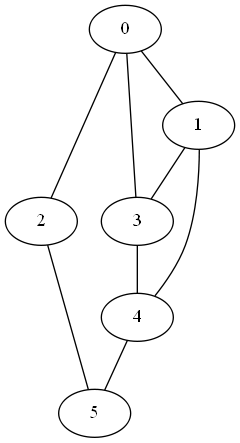The second eigenvalue of the Laplacian is 1.2679. When we connect node 2 to node 4, the second eigenvalue increases to 1.4384.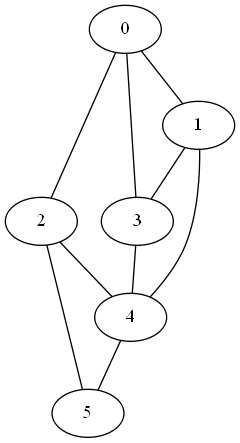If we connect node 2 to node 3 instead, the second eigenvalue goes up to 1.6972. This makes sense because node 3 is better connected than node 4. Connecting node 2 to node 3 increases the overall connectivity of the graph more than connecting it to node 4. (The second eigenvalue of the graph Laplacian is sometimes called the algebraic connectivity of the graph because it is so strongly associated with the graph’s connectivity.)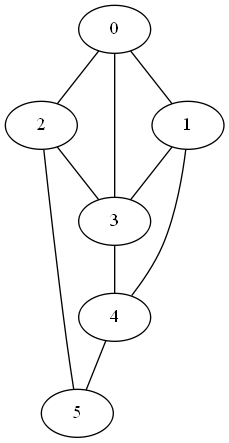Now let’s look at the rest of the eigenvalues. The full set of eigenvalues for the three graphs are given below.

original [0.0000  1.2679  2.0000  4.0000  4.0000  4.7321]
2 -> 4   [0.0000  1.4384  3.0000  4.0000  4.0000  5.5616]
2 -> 3   [0.0000  1.6972  2.3820  4.0000  4.6180  5.3028]


Notice that adding an edge between nodes 2 and 4 or between nodes 2 and 3 increased some of the eigenvalues relative to their original values and left others unchanged.

Swapping the edge between nodes 2 and 4 with an edge between nodes 2 and 3 increases the 2nd and 5th eigenvalue, but lowers the 3rd and 6th.

Related post: Social networks in fact and fiction

# Measuring connectivity with graph Laplacian eigenvalues

If a graph can be split into two components with no links between them, that’s probably easy to see. It’s also unlikely, unless there’s a good reason for it. The less obvious and more common case is a graph that can almost be split into two components. Spectral graph theory, looking at the eigenvalues of the graph Laplacian, can tell us not just whether a graph is connected, but also how well it’s connected.

The graph Laplacian is the matrix LD – A where D is the diagonal matrix whose entries are the degrees of each node and A is the adjacency matrix. The smallest eigenvalue of L, λ1, is always 0. (Why? See footnote .) The second smallest eigenvalue λ2 tells you about the connectivity of the graph. If the graph has two disconnected components, λ2 = 0. And if λ2 is small, this suggests the graph is nearly disconnected, that it has two components that are not very connected to each other. In other words, the second eigenvalue gives you a sort of continuous measure of how well a graph is connected.

To illustrate this, we’ll start with a disconnected graph and see what happens to λ2 as we add edges connecting its components.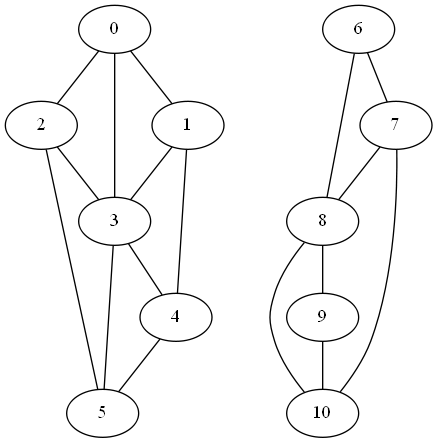The first two eigenvalues of L are zero as expected. (Actually, when I computed them numerically, I got values around 10-15, about 15 orders of magnitude smaller than the next eigenvalue, so the first two eigenvalues are zero to the limits of floating point precision.)

Next, we add an edge between nodes 4 and 9 to form a weak link between the two clusters.In this graph the second eigenvalue λ2 jumps to 0.2144.

If we connect nodes 3 and 8 instead of 4 and 8, we create a stronger link between the components since nodes 3 and 8 have more connections in their respective components. Now λ2 becomes 0.2788.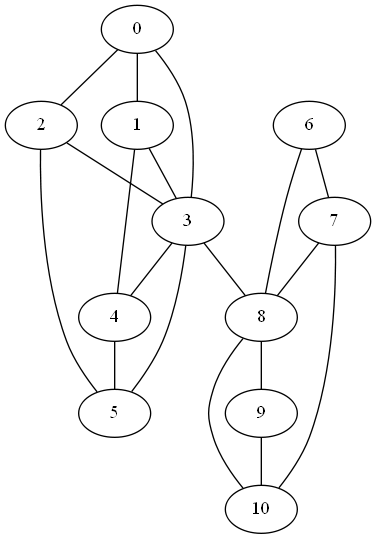Finally if we add both, connecting nodes 4 and 9 and nodes 3 and 8, λ2 increases to 0.4989.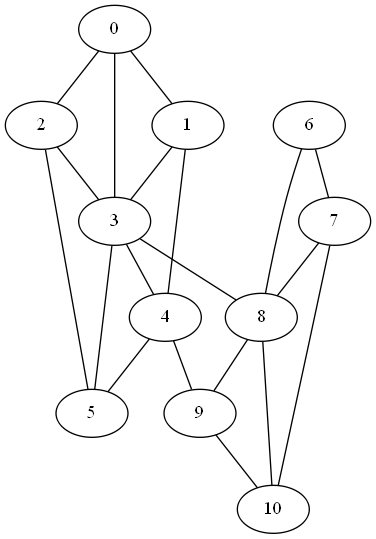## More graph theory

 To see why the smallest eigenvalue is always 0, note that v = (1, 1, 1, …, 1) is an eigenvector for 0. Multiplying the ith row of D by v picks out the degree of node i. Multiplying the ith row of A by v sums that row, which is also the degree of node i.

# Graph Laplacian and other matrices associated with a graph

There are several ways to associate a matrix with a graph. The properties of these matrices, especially spectral properties (eigenvalues and eigenvectors) tell us things about the structure of the corresponding graph. This post is a brief reference for keeping these various matrices straight.

The most basic matrix associated with a graph is its adjacency matrix, denoted A. For a graph with n nodes, create an n×n matrix filled with zeros, then fill in a 1 in the ith row and jth column if there is an edge between the ith and jth node.

Next let D be the diagonal matrix whose ith element is the degree of the ith node, i.e. the number of edges attached to the ith node. The Laplacian matrix of the graph is

L– D.

The Laplacian matrix of a graph is analogous to the Laplacian operator in partial differential equations. It is sometimes called the Kirchhoff matrix or the admittance matrix.

The signless Lapacian matrix is

Q = D.

There are connections between the signless Laplacian and bipartite components. For example, the multiplicity of 0 as an eigenvalue of Q equals the number of bipartite components in the graph.

There are other variations of the Laplacian matrix. The normalized Laplacian matrix has 1’s down the diagonal. If there is an edge between the ith and jth nodes, the ij entry is

-1/√didj

where di and dj are the degrees of the ith and jth nodes. If there is no edge between the ith and jth nodes, the ij entry is 0.

The distance matrix of a graph is the matrix D whose ijth entry is the distance from the ith node to the jth node. (Sorry, this D is different than the one above. Books often write this as a script D, something I can’t do in plain HTML.) The transmission of a node v, Tr(v), is the sum of the distances from v to every element connected to v. The distance Laplacian of a graph is Diag(Tr) – D where Diag(Tr) is the diagonal matrix whose ith entry is the transmission of the ith node and D here is the distance matrix. The signless distance Laplacian of a graph is Diag(Tr) + D.

Reference: Inequalities for Graph Eigenvalues

# Visualizing category concept dependencies

Category theory has a high ratio of definitions to theorems, and it can seem like every definition depends on an infinite regress of definitions. But if you graph the definition dependencies, the graph is wide rather than deep.

I picked out 65 concepts and created a visualization using GraphViz. No definition is more than six degrees away from first principles, and most are closer. The graph is pretty big, so here’s a thumbnail.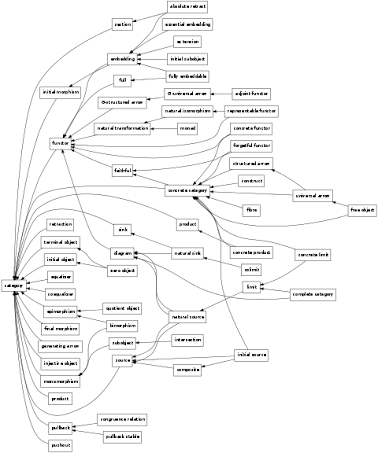The full graph is available here.

It’s possible to define concepts in different ways and obtain a different graph. I used definitions from here.

Here is a list of other math diagrams I’ve created.

Related: Applied category theory

# Stewart’s cube

This is a drawing of Stewart’s cube.  At each corner, the sum of the weights of the incoming edges is 83. Each edge has a prime weight, and all the weights are distinct.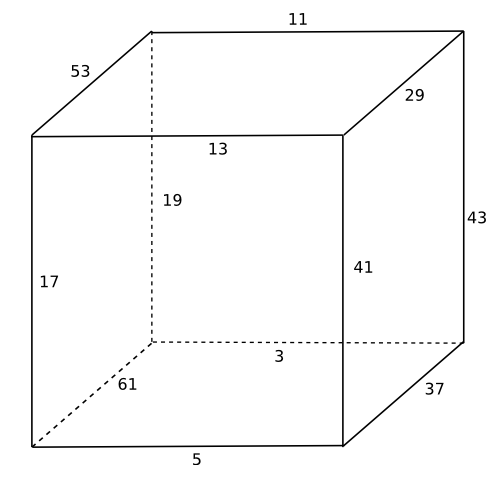Source

Update: See Austin Buchanan’s post. He found a similar cube with smaller primes.

# New Twitter account for networks

As I mentioned a few days ago, I’m winding down three of my Twitter accounts.

But I have started a new one: NetworkFact. This account has tweets about graph theory, analysis of large networks, etc.List of daily tip accounts

# Giant components and the Lambert W-function

The Lambert W-function is the function w(z) implicitly defined by w exp(w) = z. When I first saw this, I thought that I’d seen something like this come up many times and that it would be really handy to know that this function has a name and has many software implementations . Since then, however, I’ve hardly ever run into the W-function in application. But here’s an application I ran into recently.

A “giant component” in a random network is a component whose size grows in proportion to the size of the network. For a Poisson random network, let c = p(n – 1) be the expected number of vertices connected to any given vertex. If c > 1 then as n goes to infinity, there will be a giant component with probability 1. S, the proportion of nodes inside the a giant component, satisfies the equation

S = 1 – exp(-cS).

S plays a role similar to that of w in the definition of the W-function, which suggests the W-function might come in handy. And in fact, this book gives this solution for S:

S = 1 + w( –c exp(-c) )/c.

There are a couple issues. First, it’s not obvious that solution is correct. Second, we need to be more careful about how we define w(z) when z is negative.

Given some value of c, let S = 1 + w( –c exp(-c) )/c. Then

w( –c exp(-c) ) = –c(1 – S).

Apply the function f(x) = x exp( x ) to both sides of the equation above. By the definition of the W-function, we have

c exp(-c) = –c(1 – S) exp( –c(1 – S) ).

From there it easily follows that

S = 1 – exp(-cS).

Now let’s look more carefully at the definition of the W-function. For positive real z, w exp(w) = z has a unique real solution defining w. But in the calculations above, we have evaluated w at –c exp(-c), which is negative since c > 1. For negative arguments, we have to pick a branch of w. Which branch guarantees that S = 1 – exp(-cS)? Any branch . No matter which solution to w exp(w) = z we pick, the resulting S satisfies the giant component equation. However, the wrong branch might result in a meaningless solution. Since S is a proportion, S should be between 0 and 1.

Let’s go back and say that for our purposes, we will take w(z) to mean the real number no less than -1 satisfying w exp(w) = z. Then for c > 1, the solution S = 1 + w( –c exp(-c) )/c is between 0 and 1. You can show that S = 1 – exp(-cS) has a unique solution for 0 < S < 1, so any other branch would not give a solution in this interval.

Related post: Measuring graph connectivity with eigenvalues

 For example, in Python the Lambert W-function is scipy.special.lambertw. The function takes two arguments: z and an integer denoting the branch. By default, the branch argument is set to 0, giving the branch discussed in this post.

 For -1/e < z < 0, there are two real branches of w(z), but there are also infinitely many complex branches.